# Electromagnetic ClutchIt is used for the counter and the pump unit and transmit the engine rotation power to the pump.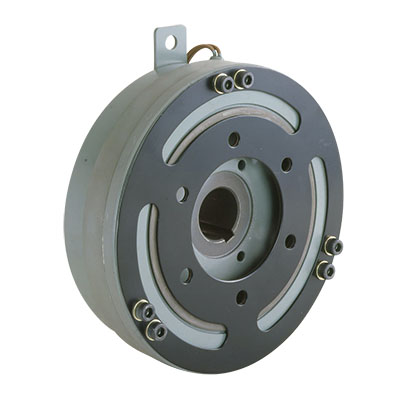## MMC-Electromagnetic Clutch

・High reliability and High torque
・Various kinds and fits to every small fishing vessels.
・One switch enables the clutch operation.
・The rotation direction of the clutch can be selected.
・High durability to the vibration and shock with a special plate spring.
・Better heat dissipation and heavy duty ones.

Electromagnetic clutch is the device to transmit the rotation power of engines as the driven power of the generator and the hydraulic pump and so on by controlling the electromagnetic power.
This can be widely used for the hydraulic pump unit and the counter assembly.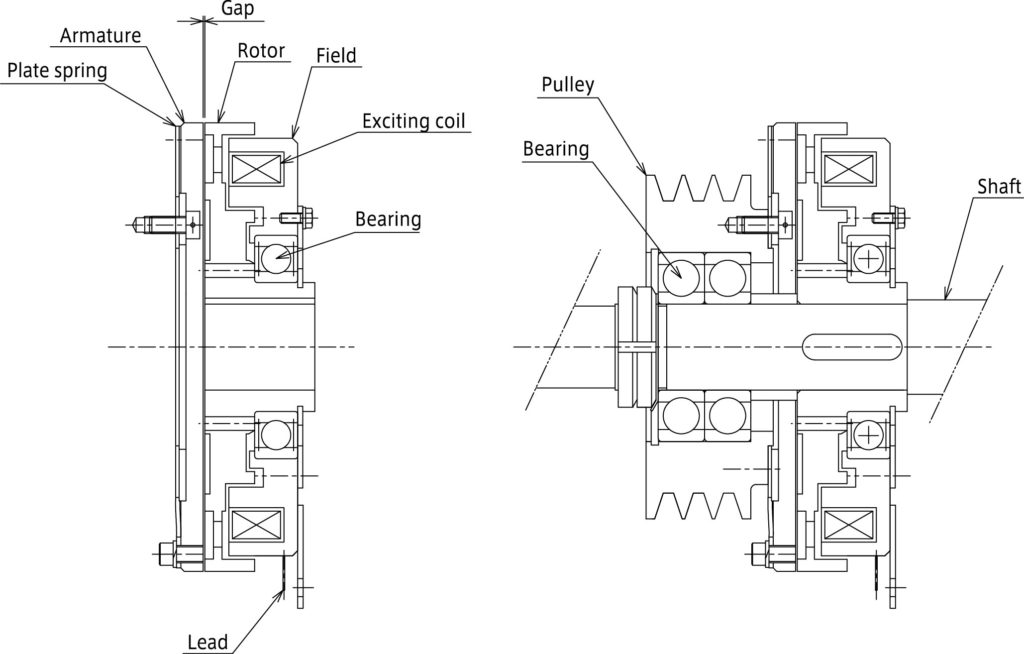Ass’y of Electromagnetic Clutch and Pulley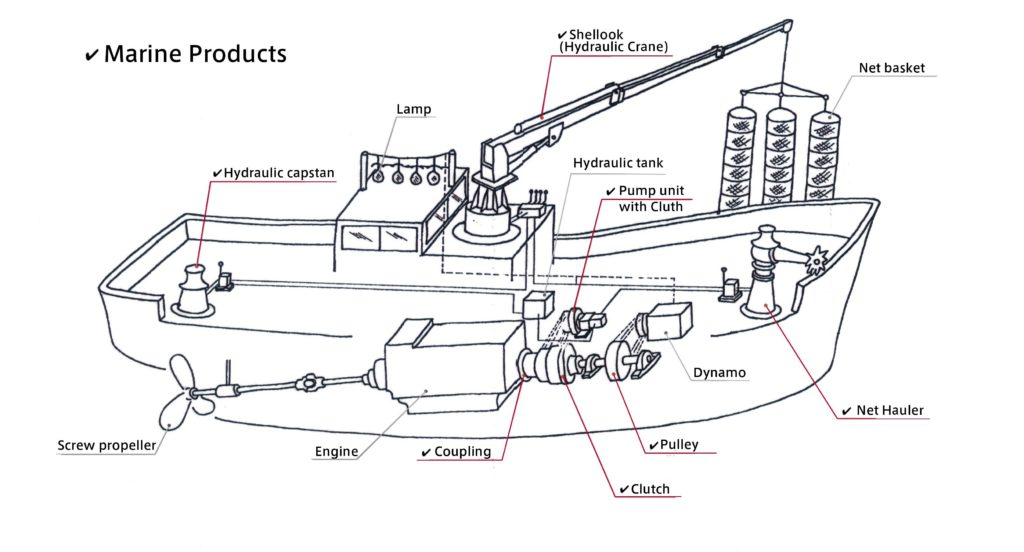SUGA’s Marine Products (✔️)

### MMC-PU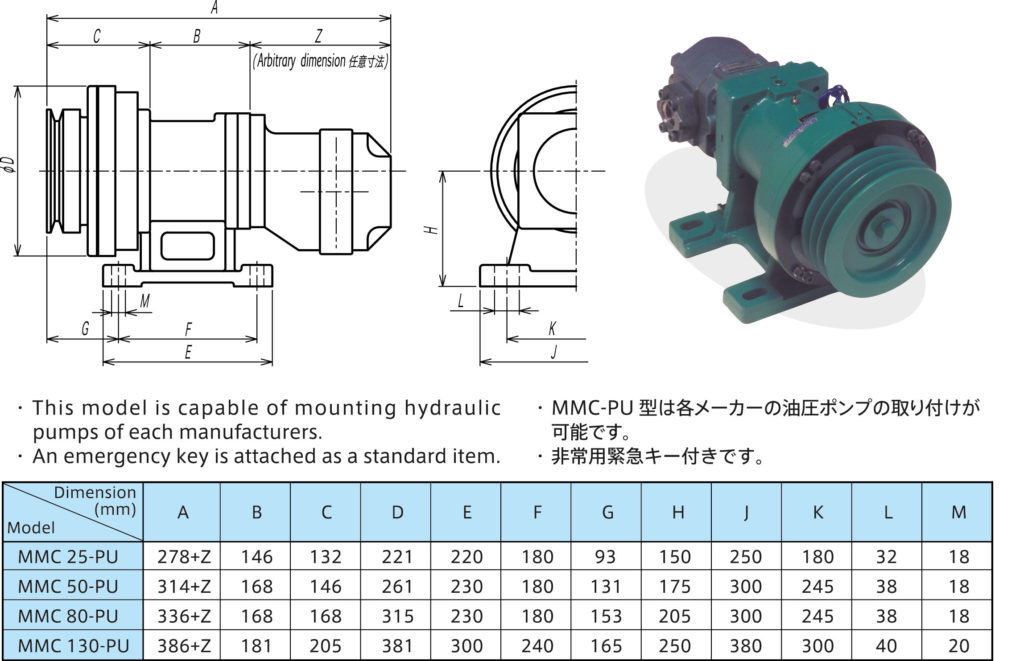### MMC-PS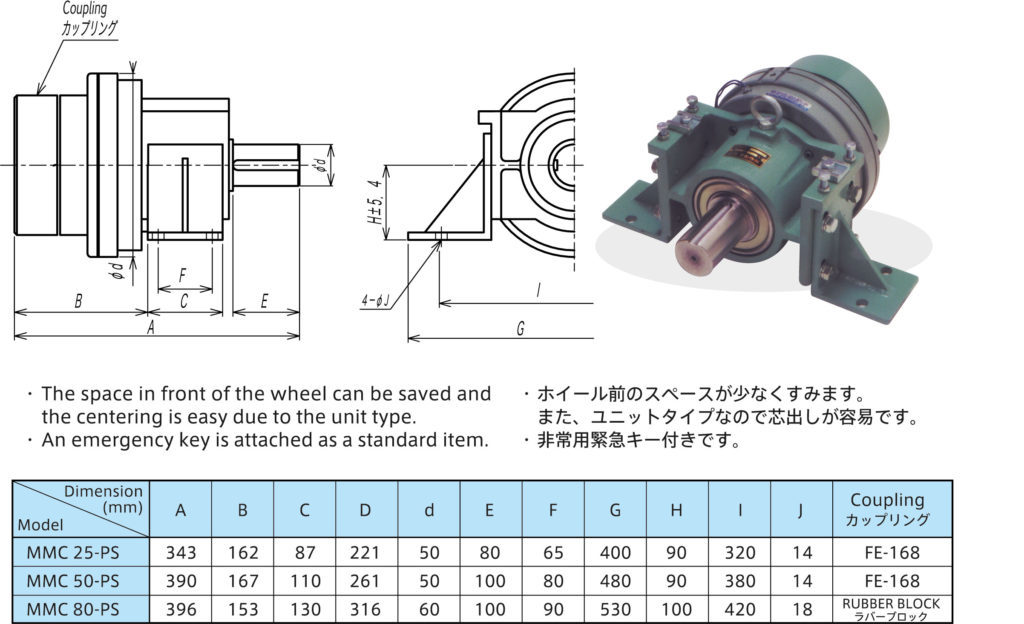### MMC-PF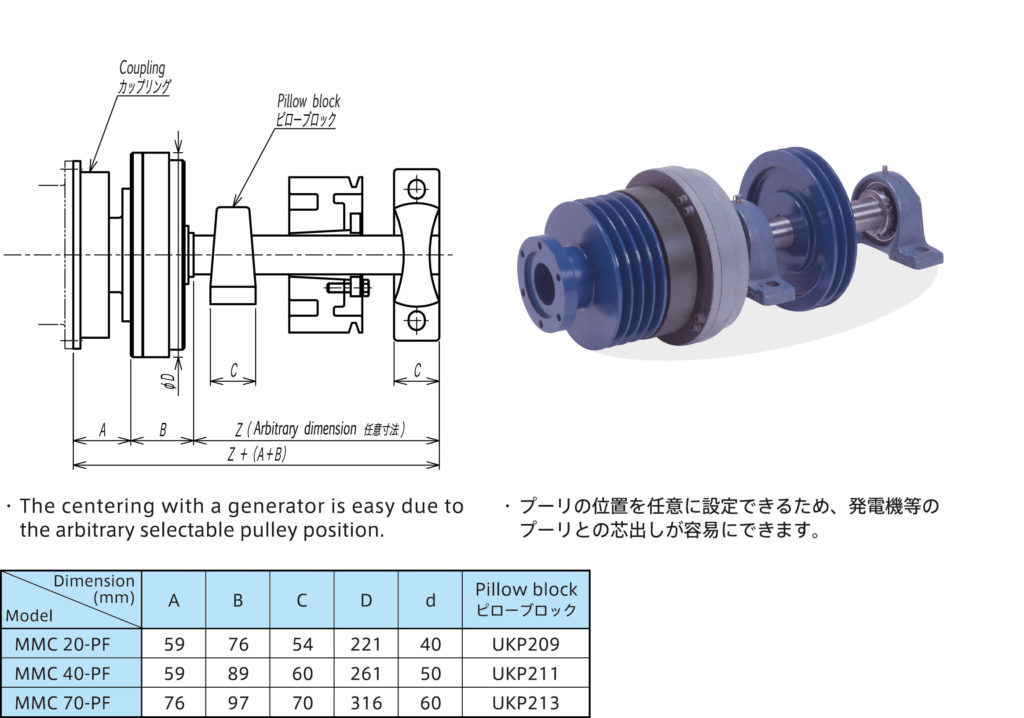### MMC-SD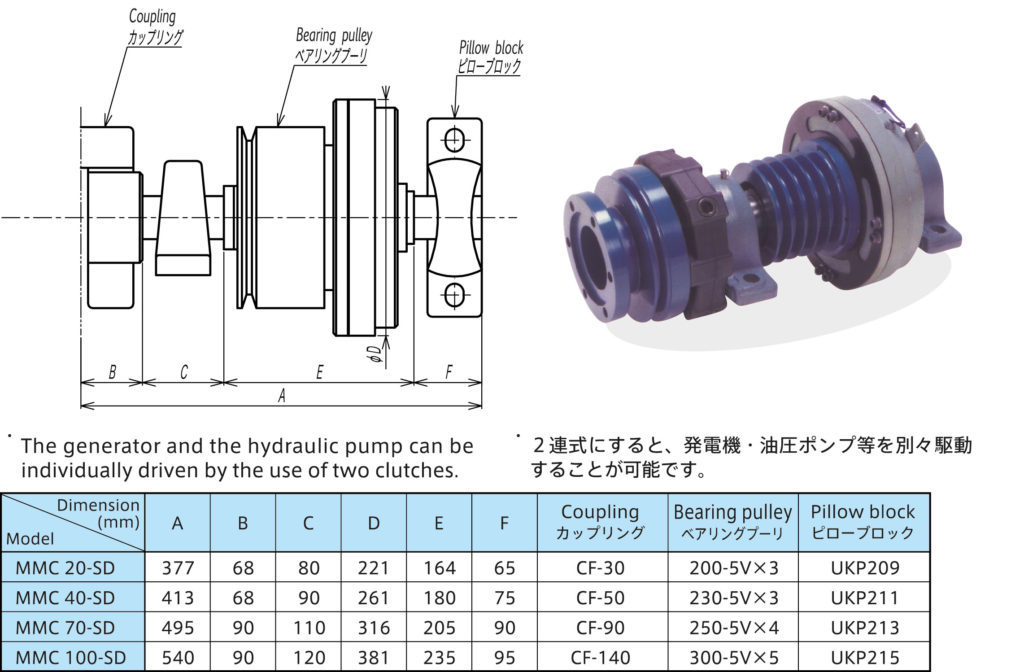#### Main Characteristics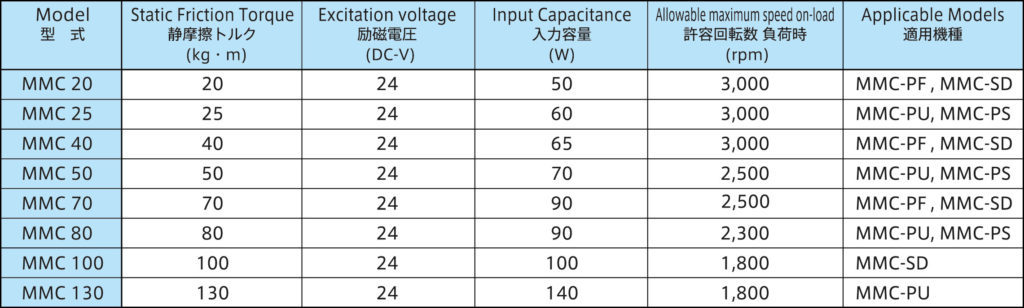#### Delivered Power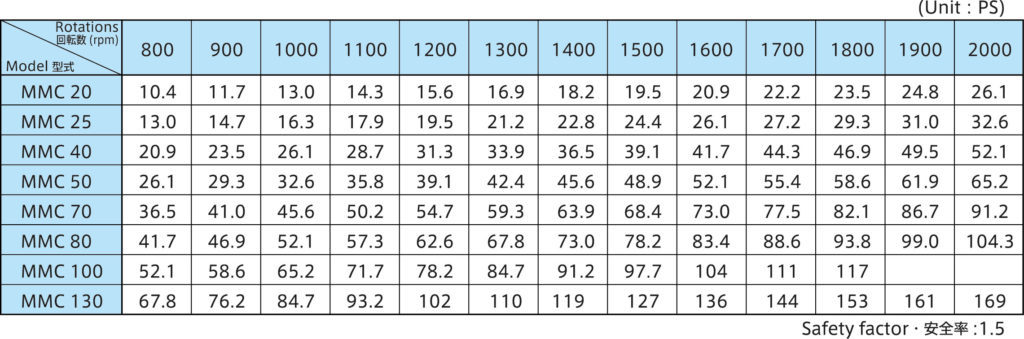#### Calculating formula for necessary oil pump power. (Applicable Model : MMC-PU)

Power ＝P×Q／450 (PS)
P：Pressure (kg/cm2)
Q：Flow (L/min)

## I　Major Calculation formula of clutch and brake

1. ### Calculation of a torque

• T=9550･P/n=7017･PS/n
2. ### Calculation of the average kinetic friction torque

• 1)　n the case of no load torque
Td=J･n/9.55tae
• 2)　In the case of the load torque
Start acceleration　Td=(J･n/9.55tae)+Tl
Deceleration stop　Td= (J･n/9.55tab)-Tl
3. ### Calculation of operate time

• 1)　n the case of no load torque.
tae=tab=2π･J･n/60Td=J･n/9.55Td
• 2)　In the case of the load torque
Start acceleration　tae=2π･J･n/60(Td-Tl)=J･n/9.55(Td-Tl)
Deceleration stop　tab=2π･J･n/60(Td+Tl)=J･n/9.55(Td+Tl)
4. ### Calculation of work

• 1)　In the case of no load torque.
Ee=Eb=J･ｎ2/182
• 2)　In the case of the load torque
Start acceleration　Ee=(J･ｎ2/182)・(Td/Td-Tl)
Deceleration stop　Eb=(J･ｎ2/182)・(Td/Td+Tl)
5. ### Calculation of the power

• 1)　when there is no load torque.
Pe=Pb=(J･ｎ2/182)・(Nc/60)
• 2)　In the case of the load torque
Start acceleration　Pe=(J･ｎ2/182)・(Td/Td-Tl)･(Nc/60)
Deceleration stop　Pb=(J･ｎ2/182)・(Td/Td+Tl)･(Nc/60)
6. ### Calculation of a life span

• L=V/w･Ee(orEb)=Et/ Ee(orEb)

### The symbol explanation

The T: torque (Nm)
P: motor output (kW)
PS: motor output (HP or hp)
The number of rotations of the
n: clutch brake shaft (r/min)
The kinetic friction torque of the
Td: Dynamic Friction Torque of clutch・brake (Nm)
J: course, the total of the moment of inertia (Kcm2)
Tae: The actual connection hour of the clutch (s)
Tab: Active braking time
Ee: Clutch connection work(J)
Eb: Braking work of brake(J)
Pe: The connection power of the clutch (W)
Pb: The braking work rate of the brake (W)
Nc: The operation frequency of the clutch・brake (time /min)
L: The connection (braking) number of times until the life (the time)
V: The general volume to the friction plate wear limit (cm3)
w: wear rate (cm3/J)
Et: Total work to the wear limit (J)

## Ⅱ　Calculation Formula of the coupling torque.

1. ### The rated torque

• T=9550･P/n=7017･PS/n

• TD=T･K

### The symbol explanation

T: rated torque (Nm)
TD: design torque (Nm)
P: motor output (kW)
PS: motor output (HP or hp)
n: The rotation number of the coupling (r/min)
K: service factor